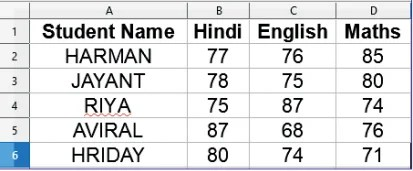Share with others

Name
Email
School Name
Q61. Do all questions by considering the following sheet. Write the formula in E2 to find the total marks scored by HARMANQ62. When you drag the formula written above from E2 to E4, then the formula becomes :

Q63. When you drag the formula written above from E2 to G2, then the formula becomes :

Q64. Write the formula/function in cell B7 to find the highest score in Hindi.

Q65. Write the formula/function in cell B8 to find the total number of students who appeared in Hindi?

Q66. Write the formula in cell B9 to find the lowest score in Hindi.

Q67. Write formula in cell E2 to calculate the percentage of Herman.(All marks are out of 100)

Q68. Write formula in cell F4 to calculate average marks of Riya.

Q69. Write formula in G5 to calculate the highest marks of Aviral.

Q70. Write formula in cell A7 to count the number of students.

Share with others
error: Content is protected !!